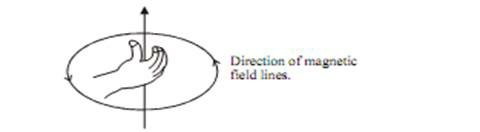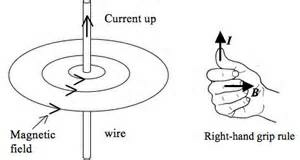# Magnetic field due to Current Carrying Conductor

The above electric circuit in which a copper wire is placed parallel to a compass needle, shows the deflection in needle gets reversed, when the direction of current reversed. Hence electricity and magnetism are related to each other.

# Right Hand Thumb Rule

It is a convenient way of finding the direction of magnetic field associated with current carrying conductor. Hold the straight wire carrying current in your right hand such that thumb points towards the direction of current, then your folded fingers around the conductor will show the direction of magnetic field.This rule also called Maxwell’s corkscrew rule.

# Magnetic Field due to Current through a Straight Conductor

• Let a current carrying conductor be suspended vertically and the electric current is flowing from south to north. In this case, the direction of magnetic field will be anticlockwise. If the current is flowing from north to south, the direction of magnetic field will be clockwise.
• A current carrying straight conductor has magnetic field in the form of concentric circles; around it. Magnetic field of current carrying straight conductor can be shown by magnetic field lines.# Magnetic Field due to Current through a circular LoopEvery point on the wire carrying current give rise to the magnetic field, appearing as a straight line at the centre of loop. By applying Right hand Thumb rule, we can find the direction of magnetic field at every section of the wire.